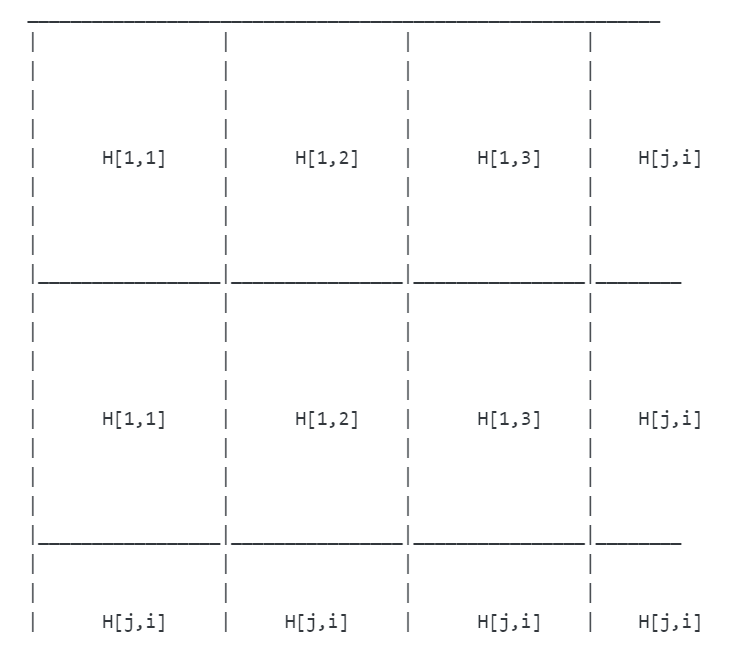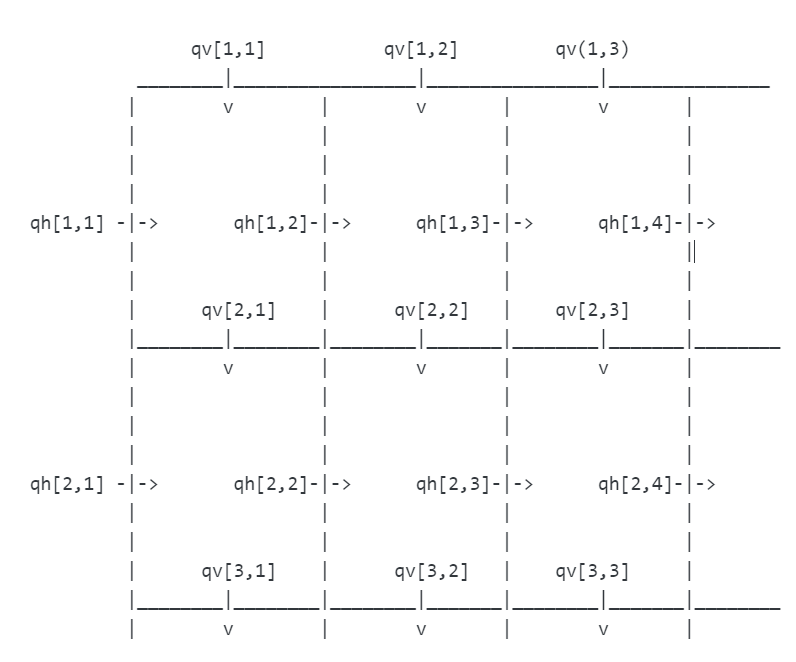Want to share your content on R-bloggers? click here if you have a blog, or here if you don't.

So far, we have learned how to count spatial variability in our model. Please look at these two previous exercises here and here if you haven’t tried it yet. However, it only represents 1-Dimension model. On this exercise, we will try to expand our spatial consideration into 2-Dimension model.

Have a look at this plan view below to get an illustration of how the 2-dimensions model will work.

The water levels are store in a 2-D array. they are numbered as follows:The water flows are store in 2 different 2-D arrays.
1. qv : defines water flows between buckets down the screen (in plan view)
2. qh : defines water flows between buckets across the screen.Let’s get into the modelling by cracking the exercises below. Answers to these exercises are available here. If you obtained a different (correct) answer than those listed on the solutions page, please feel free to post your answer as a comment on that page.

Exercise 1
Set all the required settings for the model:
a. set number of timesteps. Here we use 1000 (it shows how many timesteps in which we are going to run the model, you can change it as you want)
b. set the total number of cell, here we have 25 x 25 water tanks
c. set timestep in seconds; here is 1
d. set time at the start of simulations
e. set k between each water tank. Here we set a uniform value of k; 0.01

Exercise 2
create matrix H for initial water level in the water tank

Exercise 3
Set boundary conditions for the model; here we have water flow (qh) into the water tanks from three sides (top, left and right) and water flowing out on the bottom (qv;see the plan view). Water flow to the right and to the bottoms are considered positive. Don’t forget to declare the matrix for qh and qv.

Exercise 4
Create an output models for every 100 timesteps

Exercise 5
Run the model by creating loop for qh, qv, water storage update, and models output (Remember the threshold loop on latest previous exercise?)

Exercise 6
Plot model output using contour plot